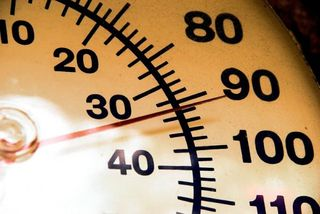# Temperature: Facts, History & DefinitionTemperature is a numerical representation of hot or cold compared against baselines, typically the point at which water freezes and boils.

In most in instances, temperature measures how much heat there is in a particular entity —in a liquid, such as water, or a solid, such as soil. In meteorology, temperature measures the heat content of the atmosphere.

The instrument used to measure temperature is a thermometer, a word derived from the Greek thermos, meaning "hot," and metron, "measure."

## History of the thermometer

Galen, a Greek scientist and physician, made the first attempt at measuring temperature in A.D. 170. He documented a standard "neutral" temperature of equal parts of boiling water and ice. He added four degrees of heat and four degrees of cold on either side of this neutral temperature.

The concept of measuring temperature is fairly new. The thermoscope — essentially a thermometer without a scale — was the precursor to the modern thermometer. There were several inventors working on thermoscopes around 1593, but the most well known is Galileo Galilei, an Italian inventor who also improved upon (but did not invent) the telescope.

A thermoscope could show the differences in temperature, allowing observers to know if something was getting hotter or colder. However, the thermoscope could not provide an exact temperature in degrees. In 1612, the Italian inventor Santorio Santorio added a numerical scale on his thermoscope and it was used to take human temperature.

Ferdinand II, the Grand Duke of Tuscany, followed in 1654, inventing the first enclosed thermometer, using alcohol as a liquid. But it still lacked a standardized scale and was not very accurate.

Around the same time, German physicist Daniel Gabriel Fahrenheit met Olaus Roemer, a Danish astronomer, who developed an alcohol-based thermometer using wine. He marked two points on his thermometer — 60 to mark the temperature of boiling water and 7.5 as the point where ice melted.

In 1714, Fahrenheit refined Roemer’s invention and developed the first modern thermometer — the mercury thermometer with more refined measurements. The mercury expands or contracts as the temperature rises or falls.

Fahrenheit had invented an alcohol thermometer in 1709 prior to unveiling its mercury counterpart, which proved to be more accurate.

Ten years later, he unveiled his namesake Fahrenheit scale, which divided the freezing and boiling points of water into 180 degrees. It established 32 degrees was the freezing pint of water and 212 degrees was the boiling point of water. An equal mixture of water, ice and ammonium chloride, a salt, was used to set the temperature of 0.

## The rise of the temperature scales

Fahrenheit is one of three major temperature scales used today, with the other two being Celsius and Kelvin. Fahrenheit is the standard used for measuring temperature in the United States, but most of the rest of the world uses Celsius.

Not too long after the Fahrenheit scale was unveiled, Swedish astronomer Anders Celsius came out with his temperature scale, which is referred to as the Celsius scale. It is sometimes called the centigrade scale, because it is divided into 100 degrees separating the boiling and freezing points of water.  The original scale set 0 as the boiling point of water and 100 as the freezing point, but he flipped it around soon after inventing the scale.

The term Celsius was adopted in 1948 by an international conference on weights and measures and the scale is the preferred temperature gauge for scientific applications as well as most of the world outside of the United States.

Lord Kelvin of Scotland chimed in with his temperature gauge in 1848, known as the Kelvin scale. He based it on the idea of absolute temperature, a theoretical temperature at which all substances have no heat energy.  There are no negative numbers on the Kelvin scale, 0 K being the coldest temperature possible.

Absolute zero converts to minus 273.15 C and minus 459.67 F. The Kelvin scale is commonly used in scientific applications. The units on the Kelvin scale are the same size as those of the Celsius scale, except that the Kelvin scale sets the lowest temperature at 0.

## Conversion rates

• Fahrenheit to Celsius: Subtract 32, then multiply by 5, then divide by 9
• Celsius to Fahrenheit: Multiply by 9, divide by 5, then add 32
• Fahrenheit to Kelvin: Subtract 32, multiply by 5, divide by 9, then add 273.15.
• Kelvin to Fahrenheit: Subtract 273.15, multiply by 1.8, then add 32.
• Kelvin to Celsius: Add 273
• Celsius to Kelvin: Subtract 273